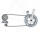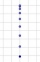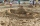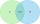Latest word problems - page 10

1. Water tankWhat is the height of the cuboid-shaped tank with the bottom dimensions of 80 cm and 50 cm if the 480 liters of water reaches 10 cm below the top?
2. Temperature changeThe mean temperature change is -3.2°F per day for five days. What is the total change over the five day period?
3. Decorative fenceThe rectangular garden is 57 m long and 42 m wide. Calculate how much m² it will reduce if it encloses with a 60 cm wide decorative fence.
4. Last pageTwo consecutive sheets dropped out of the book. The sum of the numbers on the sides of the dropped sheets is 154. What is the number of the last page of the dropped sheets?
5. Four paversFour pavers would pave the square in 18 days. How many pavers do you need to add to done work in 12 days?
6. Centroid - two bodiesA body is composed of a 0.8 m long bar and a sphere with a radius of 0.1m attached so that its center lies on the longitudinal axis of the bar. Both bodies are of the same uniform material. The sphere is twice as heavy as the bar. Find the center of gravit
7. Shopping centerThe shopping center buys from the manufacturer bikes at a purchase price of 180 €. It sells them for a sale price of 250 €. However, in the advertising for the sale of these goods, the shopping center spent 20% of the selling price of all bicycles in stock
8. PotatoesFor three days the store sold 1400 kg of potatoes. The first day they sold 100 kilograms of potatoes less than the second day, the third-day three-fifths of what they sold the first day. How many kgs of potatoes sold every day?
9. Nine booksNine books are to be bought by a student. Art books cost \$6.00 each and biology books cost \$6.50 each . If the total amount spent was \$56.00, how many of each book was bought?
10. Rocket startThe body launched vertically up returns to the start site in 6 seconds. What height did it have?
11. Sand castleTim and Tom built a sand castle and embellished it with a flag. Half the pole with the flag plunged into the castle. The highest point of the pole was 80 cm above the ground, its lowest point 20 cm above the ground. How high was the sand castle?
12. The sides 2The sides of a trapezoid are in the ratio 2:5:8:5. The trapezoid’s area is 245. Find the height and the perimeter of the trapezoid.
13. Percentage increaseIncrease number 400 by 3.5%
14. Find parametersFind parameters of the circle in the plane - coordinates of center and radius: ?
15. Prism 4 sidesFind the surface area and volume four-sided prism high 10cm if its base is a rectangle measuring 8 cm and 1.2dm
16. The workThe work was to be done by 150 workers. At the beginning of their work, their number reduced by 40, which increased the time of work by 5 and 1/3 of the schedule. How long did work take?
17. Small paintersThere are ten pupils in the painting group. Eight pupils paint with watercolors and nine pupils with tempera colors. How many pupils paint both water and tempera colors when each pupil paints?
18. LighthouseMarcel (point J) lies in the grass and sees the top of the tent (point T) and behind it the top of the lighthouse (P). | TT '| = 1.2m, | PP '| = 36m, | JT '| = 5m. Marcel lies 15 meters away from the sea (M). Calculate the lighthouse distance from the sea.
19. Three peopleThree people start doing a work at a same time. The first worked only 2 hours. The second ended 3 hours before the end. On an individual basis, it would take the first time to do the work 10 hours, second 12 hours and 15 hours third. How many hours did it.
20. Find the 9Find the missing angle in the triangle and then name triangle. Angles are: 95, 2x+15, x+3

Do you have an interesting mathematical word problem that you can't solve it? Enter it, and we can try to solve it.

To this e-mail address, we will reply solution; solved examples are also published here. Please enter e-mail correctly and check whether you don't have a full mailbox.